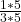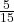## Compare the fractions using >,<, or = 3/15 and 1/3

Question

Compare the fractions using >,<, or = 3/15 and 1/3

in progress 0
6 months 2021-08-22T14:20:28+00:00 1 Answers 0 views 0

## Answers ( )

3/15 < 1/3

Step-by-step explanation:

We have two fractions, 3/15 and 1/3. The question is asking us to compare them using the less than sign <, greater than sign >, or equal sign =.

To solve this question, what we must do is try to get both fractions the same denominators, which will be done through multiplying 3 by a certain number to get to 15, then doing the same for the numerator.

So what multiplied by 3 gets us 15?

5.

Multiply both the numerator and denominator by 5.Now that they both have the same denominator, we can compare and see which is bigger.

5/15 (1/3) and 3/15.

By this we can see that 3/15 is less than 5/15.

Therefore 3/15 < 1/3